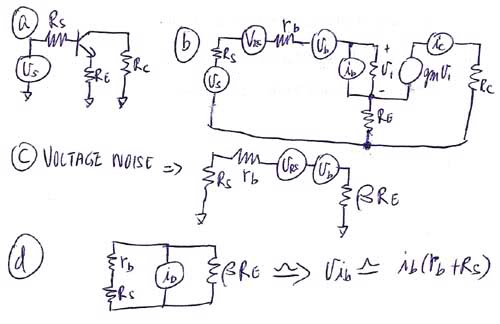# Help in noise calculation of BJT.

• yungman
In summary, Alan is trying to calculate the equivalent input noise voltage for a transistor circuit. He models the noise voltage using a simplified equivalent circuit with only base and collector resistance and neglects the noise refer from the collector current back to the input. He finds that with an emitter resistor of few kohms, the input sees the full noise voltage regardless of any R_s.

#### yungman

All the models I see is mostly common emitter, I have a circuit with AC equivalent shown in Fig a. Where $v_s \;$ is the signal voltage and the source resistance $R_s$. I want to calculate the equivalent input noise voltage. Here is what I attempt to do it:

147190[/ATTACH]"]I show the equivalent model in Fig b. I simplify by leaving the frequency depend components out for simplicity. You can see the emitter resistor and collector resistor. $r_b\;$ is the base resistance of the transistor. I left out output resistance to further simplify the circuit. $v_{RS}$ is the thermal noise of $R_s$ and $v_b \;$ is the noise voltage or the base resistance. I also left out the noise refer from the collector current back to the input by assuming gm is very high so just to look at the effect of the input components where my question is.

Fig c. show the model for calculation the equiv. input noise voltage. I assume the input resistance of the transistor is $\beta R_E\;$ where $\beta\;$ is the current gain of the transistor. As you can see $\beta R_E\;$ is much larger than $r_b+R_s\;$ therefore the input see the full $v_b+v_{RS}$.

Fig d is where my confusion. how do I model the input resistance. I attempt to use Fig d. but I am not sure this is correct.

Can anyone verify Fig c and Fig d?

So the total noise voltage:

$$v_{n_{in}}=\sqrt{ v_{RS}^2 + v_b^2+v_{ib}^2}$$

Thanks

Alan

#### Attachments

Last edited:
Also I am confused with the data sheet of the MPSA18:

http://pdf1.alldatasheet.com/datasheet-pdf/view/5139/MOTOROLA/MPSA18.html

You can see in Fig 1, the equivalent noise voltage and current is switch from what I am using which I got from textbooks. I understand it is the total equivalent noise voltage, but I still think it should be switch and voltage always has to be in series with the source and input resistance and current is parallel. You can verify by looking at any of the BJT models.

Also, it seems that Fig.2 to Fig.7 are only true for ground emitter only, if you have an emitter resistor that is over 5K, nothing apply anymore! I think the only way to use the data sheet is to find $r_b\;$ from the data sheet using Fig.2 where the source resistance is zero to get rid of the $i_b\;$ term and $e_n=v_b\;$ and use the formula

$$v_b^2=4kT\;r_b \Delta f$$

to find the base resistance and use my formulas to calculate the voltage equivalent noise( I include flicker noise into the model and just assume base resistance increase at low frequency!...Lazy!). and use $\beta\;$ to calculate equivalent noise current $i_b\;$ using the collector current by

$$i_b^2=2q\frac {I_c }{\beta} \Delta f$$

Help!

Last edited:
Anyone?

If I am correct in my assumption in Fig. c and Fig. d, this mean with the emitter resistor $R_E\;$ of a few kohms, and keep the $R_s\;$ very low, you eliminate the current noise. As for the voltage noise, $\beta R_E\;$ is so high that you always see the full noise voltage at the input regardless of any $R_s\;$ if the value is under 50K or so.

An emitter resistor reduces the gain for both the source signal and noise, but it introduces another noise source in parallel with the original, thus degrading the signal to noise ratio.

Studiot said:
An emitter resistor reduces the gain for both the source signal and noise, but it introduces another noise source in parallel with the original, thus degrading the signal to noise ratio.

Thanks for the reply. Do you have any link on this, how to model this?

Noise in Transistor Circuits

Wireless World

Article 1 November 1968 (5 pages)
Article 2 December 1968 (6 pages) This is really the important one from your point of view.

I have a rather poor photocopy of these available.

Thanks, I have not been able to find the articles, I just order the Integrated Circuit design by Paul Grey( for less than \$10! on ebay). I used to use this book when I was designing bipolar ICs, I since lost it and I remember it has a lot of models for transistors. But I read some where that there are new models like Van der Ziel model which I know nothing. The one I posted was the Grey and Meyer model.

Van Der Ziel wrote the original articles in the 1950s and one book

'Fluctuation Phenomena in Semiconductors'

Butterworths 1959

I forgot to mention the author of my previous reference - Peter Baxandell of tone control and other audio innovations fame. A PM with an email address capable of receiving jpgs gets you a scan.

go well